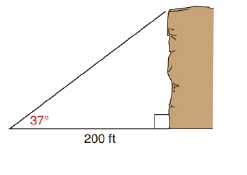Chapter 11.2, Problem 32E### Elementary Geometry for College St...

6th Edition
Daniel C. Alexander + 1 other
ISBN: 9781285195698

#### Solutions

Chapter
Section### Elementary Geometry for College St...

6th Edition
Daniel C. Alexander + 1 other
ISBN: 9781285195698
Textbook Problem
188 views

# In Exercise 29 to 37, angle measures should be given to the nearest degree; distances should be given to the nearest tenth of a unit.At a point 200 ft. from the base of a cliff, Journey sees the top of the cliff through an angle of elevation of 37 ∘ . How tall is the cliff?To determine

To find:

To find the height of the cliff by using the following condition,

“At a point 200 ft. from the base of a cliff, Journey sees the top of the cliff through an angle of elevation of 37.”

Explanation

Consider the following figure,

General formula for cosine ratio and sin ratio is given below,

From the given figure the value x ft. is the hypotenuse value since it is opposite to right angle.

Then y ft. is the length of the leg opposite to 37 and 200 ft. is the length of the leg adjacent to 37.

Therefore,

cos37=200xx=200cos37

Using a graphing calculator in degree mode, follow the following key sequence,

cos(37)enter0.7986

Substitute the value of cos37 in the above equation to get the following,

### Still sussing out bartleby?

Check out a sample textbook solution.

See a sample solution

#### The Solution to Your Study Problems

Bartleby provides explanations to thousands of textbook problems written by our experts, many with advanced degrees!

Get Started

#### Find more solutions based on key concepts